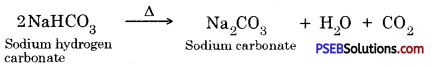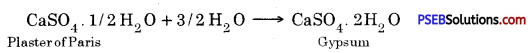# PSEB 10th Class Science Solutions Chapter 2 Acids, Bases and Salts

Punjab State Board PSEB 10th Class Science Book Solutions Chapter 2 Acids, Bases and Salts Textbook Exercise Questions and Answers.

## PSEB Solutions for Class 10 Science Chapter 2 Acids, Bases and Salts

PSEB 10th Class Science Guide Acids, Bases and Salts Textbook Questions and Answers

Question 1.
A solution turns red litmus blue, its pH is likely to be :
(a) 1
(b) 4
(c) 5
(d) 10.
(d) 10.

Question 2.
A solution reacts with crushed egg-shells to give a gas that turns lime- water milky. The solution contains:
(a) NaCl
(b) HCl
(c) LiCl
(d) KC1.
(b) HCl

Question 3.
10 mL of a solution of NaOH is found to be completely neutralised by 8 mL of a given solution of HCl. If we take 20 mL of the same solution of NaOH, the amount of HCl solution (the same solution as before) required to neutralise it will be :
(a) 4 mL
(b) 8 mL
(c) 12 mL
(d) 16 mL.
(d) 16 mL.

Question 4.
Which one of the following types of medicines is used for treating indigestion?
(a) Antibiotic
(b) Analgesic
(c) Antacid
(d) Antiseptic.
(c) Antacid

Question 5.
Write word equations and then balance equations for the reaction taking place when :
(a) dilute sulphuric acid reacts with zinc granules
Zinc granules + Dilute sulphuric acid → Zinc sulphate + Hydrogen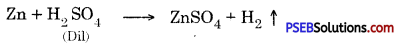(b) dilute hydrochloric acid reacts with magnesium ribbon
Magnesium ribbon + Hydrochloric acid → Magnesium chloride + Hydrogen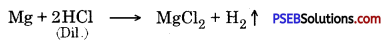(c) dilute sulphuric acid reacts with aluminium powder
Aluminium powder + Dilute sulphuric acid → Aluminium sulphate + Hydrogen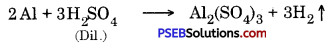(d) dilute sulphuric acid reacts with iron filings.
Iron filings + Dilute sulphuric acid → Iron (II) sulphate + Hydrogen.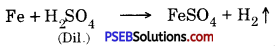Question 6.
Compounds such as alcohols and glucose also contain hydrogen but are not categorised as acids. Describe an activity to prove it.
Fix two nails on a cork and place it in a 100 ml beaker. Connect these nails to a 6 volt battery through a bulb and switch as shown in the figure.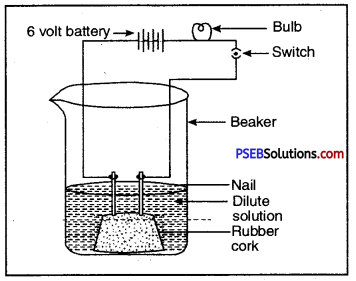Aqueous solution of alcohol or glucose does not conduct electricity

Pour some aqueous solution of alcohol or aqueous solution of glucose in the beaker so that nails dip in it. Switch on the current. The bulb does not glow indicating that alcohol and glucose don’t dissociate in aqueous solution and hence
do not produce H+ ions although they (aq)
contain hydrogen.

Question 7.
Why does not distilled water conduct electricity, whereas rainwater does?

• Distilled water does not conduct electricity because it contains no ions.
• Rainwater contains ions due to dissolved salts, hence it conducts electric current.

Question 8.
Why do acids not show acidic behaviour in the absence of water?
This is because in the absence of water, acids do not dissociate to give hydrogen ions > H+(aq)

Question 9.
Five solutions A, B, C, D and E when tested with universal indicator showed pH as 4, 1, 11, 7 and 9 respectively. Which solution is :
(a) neutral?
(b) strongly alkaline?
(c) strongly acidic?
(d) weakly acidic?
(e) weakly alkaline?
Arrange the pH in increasing order of hydrogen-ion concentration.

 Solution pH Nature of Solution A 4 Weakly acidic B 1 Strongly acidic C 11 Strongly alkaline D 7 Neutral E 9 Weakly alkaline

The increasing order of hydrogen-ion concentration is :
11 < 9 < 7 < 4 < 1 (pH values).

Question 10.
Equal lengths of magnesium ribbons are taken in test tubes A and B. Hydrochloric acid (HCl) is added to test tube A, while acetic acid (CH3COOH) is added to test tube B. Amount and concentration taken for both the acids are same. In which test tube will the fizzing occur more vigorously and why?
Fizzing occurs more vigorous in test tube A as compared to in test tube B. This is because concentration of hydrogen ion, It is more in test tube A than in test tube B, as hydrochloric acid a strong acid and acetic acid (CH3COOH) is a weak acid.

Question 11.
Fresh milk has a pH of 6. How do you think the pH will change as it turns into curd? Explain your answer.
Its pH will decrease due to the production of lactic acid which is acidic in nature.

Question 12.
A milkman adds a very small amount of baking soda to fresh milk.
(a) Why does he shift the pH of the fresh milk from 6 to slightly alkaline?
So that the milk is not spoiled readily and medium remains basic.

(b) Why does this milk take a long time to set as curd?
Because the lactic acid produced during curding reacts with baking soda.

Question 13.
Plaster of Paris should be stored in a moisture-proof container. Explain why?
This is because in presence of moisture, plaster of Paris sets to give a hard mass.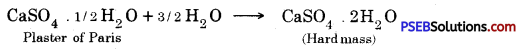Question 14.
What is a neutralisation reaction? Give two examples.
The interaction of an acid with a base to form salt and water is called neutralisation reaction.

Examples :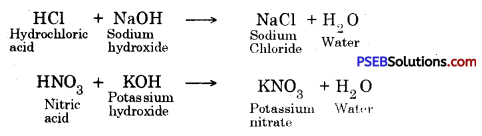Question 15.
Give two important uses of washing soda and baking soda.
(a) Uses of washing soda :

1. It is used in the manufacture of glass and soap.
2. It is used in the manufacture of borax.

(b) Uses of baking soda :

1. It is used in soda-acid fire extinguisher.
2. It is used for making baking powder.

Science Guide for Class 10 PSEB Acids, Bases and Salts InText Questions and Answers

Question 1.
You have been provided with three test tubes. One of them contains distilled water and the other two contain an acidic solution and a basic solution, respectively. If you are given only red litmus paper, how will you identify the contents of each test tube?
Dip red litmus paper in solution repeatedly in each tube.
(a) The tube in which the red litmus paper turns purple contains distilled water.
(b) The tube in which red litmus paper turns blue contains basic solution.
(c) The tube in which red litmus paper remains red contains acidic solution.

Question 2.
Why should curd and sour substances not be kept in brass and copper vessels?
Curd and other sour substances contain acids which react with the metal surface of brass and copper vessels to produce toxic compounds which are unfit for consumption.Question 3.
Which gas is usually liberated when an acid reacts with a metal? Illustrate with an example. How will you test for the presence of this gas?
When an acid reacts with metal, generally hydrogen is produced.
e.g. Mg + 2 HCl(Dil) → MgCl2 + H2

Pass this gas (H2) through soap solution. The soap bubbles filled with the gas will rise up. If a burning splinter is brought near the gas, the bubble will burn with a ‘pop’ sound.

Question 4.
Metal compound A reacts with dilute hydrochloric acid to produce effervescence. The gas evolved extinguishes a burning candle. Write a balanced chemical equation for the reaction if one of the compounds formed is calcium chloride.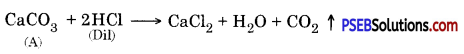CO2 extinguishes a burning candle.

Question 5.
Why do HCl, HNO3 etc. show acidic characters in aqueous solutions while solutions of compounds like alcohol and glucose do not show acidic character?
HCl, HNO3 etc undergo dissociation in water to give Hydrogen ions, H+(aq) ions and show acidic characteristics. There are compounds like alcohol and glucose don’t dissociate in water to give hydrogen ions, H+(aq) ions. Hence, they don’t show acidic properties.

Question 6.
Why does an aqueous solution of acid conduct electricity?
The aqueous solution of an acid contains ions such as hydrogen ions, H+(aq) and other anions. Hence it conducts electricity.

Question 7.
Why does dry HCl gas not change the colour on the dry litmus paper?
This is because dry HCl gas is a covalent compound and it does not undergo dissociation to give hydrogen ions, H+(aq) and hence no change in colour of dry litmus paper.

Question 8.
While diluting an acid, why is it recommended that the acid should be added to water and not water to the acid?
The process of dissolving an acid or a base in water is an exothermic process. This is because if water is added to concentrated acid, the heat generated may cause the mixture to splash out and cause burns. The glass container may also break due to excessive local heating.

Question 9.
How is the concentration of hydronium ions (H3O+) affected when a solution of an acid is diluted?
The concentration of hydronium ions decreases when a solution of an acid is diluted.

Question 10.
How is the concentration of hydroxide ions [OH] affected when excess base is dissolved in a solution of sodium hydroxide?
When excess of base is dissolved in a solution of sodium hydroxide, the concentration of OH ions increases.

Question 11.
You have two solutions, A and B. The pH of solution A is 6 and pH of solution B is 8. Which solution has more hydrogen ion concentration? Which of this is acidic and which one is basic?
In solution A, [H+(aq)] = 10-6 M, pH < 7
In solution B, [H+(aq)] = 10-8 M. pH > 7

∴ Then the solution A has more hydrogen ion concentration.
Solution A is acidic.
Solution B is basic.

Question 12.
What effect does the concentration of H+(aq) ions have on the nature of the solution?

• If [H+] < 10-7 M, it is basic solution.
• If [H+] >10-7 M, it is an acidic solution.
• If [H+] = 10-7 M, it is a neutral aqueous solution.

Question 13.
Do basic solutions also have H+(aq) ions? If yes, then why are these basic?
Basic solutions also contain H+(aq) ions. But in basic solutions :
[H+(aq)] < 10-7 M
and [OH] > 10-7 M
Since [OH(aq)] is more than [H+(aq)], hence these are basic solutions.Question 14.
Under what soil condition do you think a farmer would treat the soil of his Helds with quick lime (calcium oxide) or slaked lime (calcium hydroxide) or chalk (calcium carbonate)?
If the pH of the soil is less than 7, i.e. it is acidic, the farmer will treat the soil with quick lime, slaked lime, chalk.

Question 15.
What is the common name of the compound CaOCl2?
Bleaching powder.

Question 16.
Name the substance which on treatment with chlorine yields bleaching powder.
Dry slaked lime.

Question 17.
Name the sodium compound which is used for softening hard water.